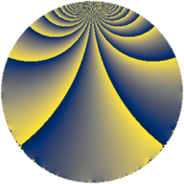Properties

 Label 84.2.eLevel $84$ Weight $2$ Character orbit 84.e Rep. character $\chi_{84}(71,\cdot)$ Character field $\Q$ Dimension $12$ Newform subspaces $1$ Sturm bound $32$ Trace bound $0$

Related objects

Defining parameters

 Level: $$N$$ $$=$$ $$84 = 2^{2} \cdot 3 \cdot 7$$ Weight: $$k$$ $$=$$ $$2$$ Character orbit: $$[\chi]$$ $$=$$ 84.e (of order $$2$$ and degree $$1$$) Character conductor: $$\operatorname{cond}(\chi)$$ $$=$$ $$12$$ Character field: $$\Q$$ Newform subspaces: $$1$$ Sturm bound: $$32$$ Trace bound: $$0$$

Dimensions

The following table gives the dimensions of various subspaces of $$M_{2}(84, [\chi])$$.

Total New Old
Modular forms 20 12 8
Cusp forms 12 12 0
Eisenstein series 8 0 8

Trace form

 $$12 q - 4 q^{4} - 6 q^{6} - 4 q^{9} + O(q^{10})$$ $$12 q - 4 q^{4} - 6 q^{6} - 4 q^{9} + 4 q^{10} - 6 q^{12} + 4 q^{16} - 8 q^{18} - 16 q^{22} + 2 q^{24} - 12 q^{25} + 8 q^{28} + 20 q^{30} - 16 q^{33} + 32 q^{34} - 20 q^{36} - 16 q^{37} + 20 q^{40} + 10 q^{42} + 24 q^{45} + 46 q^{48} - 12 q^{49} - 28 q^{52} + 10 q^{54} + 16 q^{57} - 32 q^{58} + 28 q^{60} - 16 q^{61} + 20 q^{64} - 12 q^{66} - 24 q^{69} - 12 q^{70} - 32 q^{72} + 24 q^{73} - 60 q^{76} + 20 q^{78} + 28 q^{81} + 8 q^{82} - 14 q^{84} + 40 q^{85} - 56 q^{88} - 80 q^{90} + 24 q^{93} - 34 q^{96} - 24 q^{97} + O(q^{100})$$

Decomposition of $$S_{2}^{\mathrm{new}}(84, [\chi])$$ into newform subspaces

Label Dim. $$A$$ Field CM Traces $q$-expansion
$a_{2}$ $a_{3}$ $a_{5}$ $a_{7}$
84.2.e.a $12$ $0.671$ 12.0.$$\cdots$$.2 None $$0$$ $$0$$ $$0$$ $$0$$ $$q+\beta _{4}q^{2}-\beta _{9}q^{3}+(-\beta _{2}-\beta _{3})q^{4}+\cdots$$